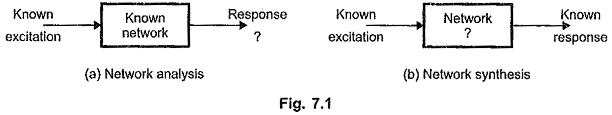## Elements of Network Synthesis:

Elements of Network Synthesis – For any network, three things are associated with it. These are network elements, input i.e. excitation to the network and output i.e. response from the network.

In the network analysis, the network elements are known and excitation is also known. Using number of methods, the networks are studied and the response is obtained. Such a response is unique for a given network and known excitation. So obtaining a response for a known network and known excitation is called network analysis.

In the network synthesis, the procedure is exactly opposite to the analysis. The excitation is known and the response requirements are known. It is necessary to find the network satisfying the requirements. Thus obtaining a network for a known excitation and known response requirements is called network synthesis.

The basic difference between network analysis and synthesis is shown in the Fig. 7.1 (a) and (b).Another important difference between analysis and synthesis is that the analysis gives the solution i.e. response which is always unique. But synthesis may give different solutions i.e. networks satisfying the required specifications. The synthesis does not give us unique solution. Sometimes it may not give the solution.

For example, the specification of the network is that its impedance Z(s) must be 10 Ω. Synthesizing of this specification may give the network with only one 10 Ω resistance or two 5 Ω resistances in series or two 20 Ω resistances in parallel and so on. So synthesis solution is not unique.

Similarly specification like Z(s) = —10 Ω can not be satisfied using passive Elements of Network Synthesis. Thus synthesis may not give any solution also.

We have seen that driving point impedance and admittance functions for all the networks, consisting of passive elements are rational and in the form of ratio of two polynomials in ‘s’. But it is not possible that every ratio of two polynomials in ‘s’ can be synthesized with Elements of Network Synthesis.

Scroll to Top